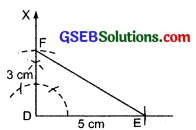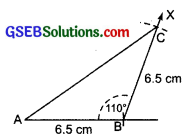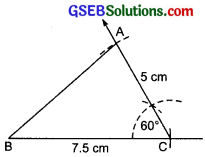# GSEB Solutions Class 7 Maths Chapter 10 Practical Geometry Ex 10.3

Gujarat Board GSEB Textbook Solutions Class 7 Maths Chapter 10 Practical Geometry Ex 10.3 Textbook Questions and Answers.

## Gujarat Board Textbook Solutions Class 7 Maths Chapter 10 Practical Geometry Ex 10.3Question 1.
Construct ∆DEF such that DE = 5 cm, DF = 3 cm and m∠EDF = 90°.
Solution:
Steps of construction:
I. Draw a line segment DE = 5 cm.
II. AT D, construct ∠EDX = 90°.
III. From the ray DX, cut off DF = 3 cm.
IV. Join EF.Then ∆DEF is the required triangle.

Question 2.
Construct an isosceles triangle in which the lengths of each of its equal sides is 6.5 cm and the angle between them is 110°.
Solution:
Steps of construction:
I. Draw a line segment AB = 6.5 cm.
II. At B, using a protractor construct ∠ABX = 110°.
III. From the ray BX, cut off BC = 6.5 cm.
IV. Join CA.Thus, ∆ABC is the required isosceles triangle.Question 3.
Construct ∆ABC with BC = 7.5 cm, AC = 5 cm and m∠C = 60°.
Solution:
Steps of construction:
I. Draw a line segment BC = 7.5 cm.
II. At C, construct ∠BCX = 60°.
III. From the ray CX, cut off CA = 5 cm.
IV. Join AB.Thus, ∆ABC is the required triangle.